### Home > CAAC > Chapter 7 > Lesson 7.1.4 > Problem7-40

7-40.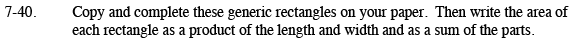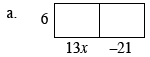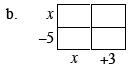To write the area as a sum:

Find the area of each of the rectangle's sections. Then, add them together.

To write the area as a product:

Multiply the total length by the total width, but don't simplify it.

As a sum: 78x−126
As a product: 6(13x−21)

See the help for part (a).

As a sum: x²−2x−15
As a product: (x−5)(x+3)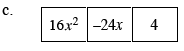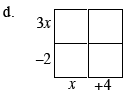Find the greatest common factor of the areas. That will be the width. Then divide each area by the width to find the length. Then follow the steps for part (a).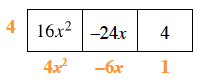See the help for part (b).